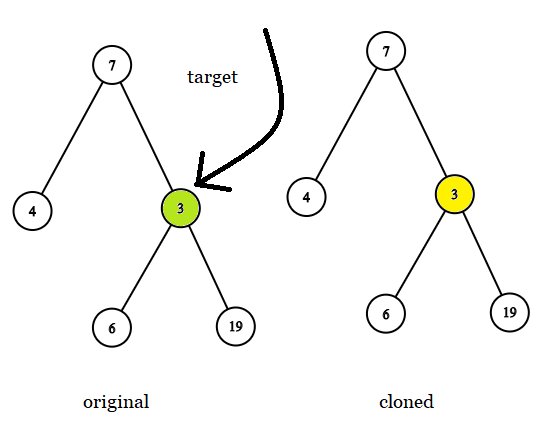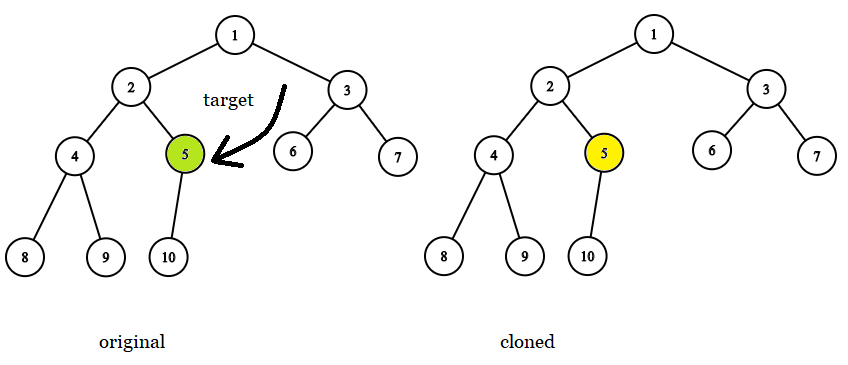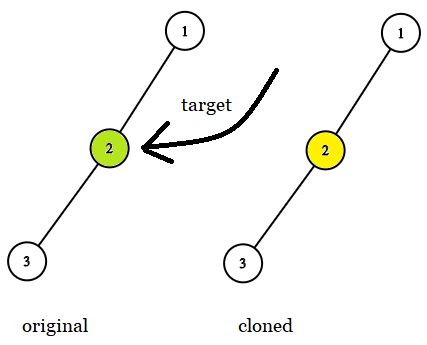##### Welcome to Subscribe On Youtube

Formatted question description: https://leetcode.ca/all/1379.html

# 1379. Find a Corresponding Node of a Binary Tree in a Clone of That Tree (Medium)

Given two binary trees original and cloned and given a reference to a node target in the original tree.

The cloned tree is a copy of the original tree.

Return a reference to the same node in the cloned tree.

Note that you are not allowed to change any of the two trees or the target node and the answer must be a reference to a node in the cloned tree.

Follow up: Solve the problem if repeated values on the tree are allowed.

Example 1:Input: tree = [7,4,3,null,null,6,19], target = 3
Output: 3
Explanation: In all examples the original and cloned trees are shown. The target node is a green node from the original tree. The answer is the yellow node from the cloned tree.


Example 2:Input: tree = , target =  7
Output: 7


Example 3:Input: tree = [8,null,6,null,5,null,4,null,3,null,2,null,1], target = 4
Output: 4


Example 4:Input: tree = [1,2,3,4,5,6,7,8,9,10], target = 5
Output: 5


Example 5:Input: tree = [1,2,null,3], target = 2
Output: 2


Constraints:

• The number of nodes in the tree is in the range [1, 10^4].
• The values of the nodes of the tree are unique.
• target node is a node from the original tree and is not null.

Related Topics:
Tree

## Solution 1.

• /**
* Definition for a binary tree node.
* public class TreeNode {
*     int val;
*     TreeNode left;
*     TreeNode right;
*     TreeNode(int x) { val = x; }
* }
*/

class Solution {
public final TreeNode getTargetCopy(final TreeNode original, final TreeNode cloned, final TreeNode target) {
TreeNode copy = null;
queue1.offer(original);
queue2.offer(cloned);
while (!queue1.isEmpty()) {
TreeNode node1 = queue1.poll();
TreeNode node2 = queue2.poll();
if (node1 == target) {
copy = node2;
break;
}
TreeNode left1 = node1.left, right1 = node1.right;
TreeNode left2 = node2.left, right2 = node2.right;
if (left1 != null) {
queue1.offer(left1);
queue2.offer(left2);
}
if (right1 != null) {
queue1.offer(right1);
queue2.offer(right2);
}
}
return copy;
}
}

############

/**
* Definition for a binary tree node.
* public class TreeNode {
*     int val;
*     TreeNode left;
*     TreeNode right;
*     TreeNode(int x) { val = x; }
* }
*/

class Solution {
private TreeNode res;

public final TreeNode getTargetCopy(
final TreeNode original, final TreeNode cloned, final TreeNode target) {
dfs(original, cloned, target);
return res;
}

private void dfs(TreeNode original, TreeNode cloned, TreeNode target) {
if (cloned == null) {
return;
}
if (original == target) {
res = cloned;
return;
}
dfs(original.left, cloned.left, target);
dfs(original.right, cloned.right, target);
}
}

• // OJ: https://leetcode.com/problems/find-a-corresponding-node-of-a-binary-tree-in-a-clone-of-that-tree/
// Time: O(N)
// Space: O(H)
class Solution {
public:
TreeNode* getTargetCopy(TreeNode* original, TreeNode* cloned, TreeNode* target) {
if (!original) return nullptr;
if (original == target) return cloned;
auto ans = getTargetCopy(original->left, cloned->left, target);
return ans ? ans : getTargetCopy(original->right, cloned->right, target);
}
};

• # Definition for a binary tree node.
# class TreeNode:
#     def __init__(self, x):
#         self.val = x
#         self.left = None
#         self.right = None

class Solution:
def getTargetCopy(
self, original: TreeNode, cloned: TreeNode, target: TreeNode
) -> TreeNode:
res = None

def dfs(original, cloned):
nonlocal res
if cloned is None:
return
if original == target:
res = cloned
return
dfs(original.left, cloned.left)
dfs(original.right, cloned.right)

dfs(original, cloned)
return res


• /**
* Definition for a binary tree node.
* class TreeNode {
*     val: number
*     left: TreeNode | null
*     right: TreeNode | null
*     constructor(val?: number, left?: TreeNode | null, right?: TreeNode | null) {
*         this.val = (val===undefined ? 0 : val)
*         this.left = (left===undefined ? null : left)
*         this.right = (right===undefined ? null : right)
*     }
* }
*/

function getTargetCopy(
original: TreeNode | null,
cloned: TreeNode | null,
target: TreeNode | null,
): TreeNode | null {
if (cloned === null) {
return null;
}
if (cloned.val === target.val) {
return cloned;
}
return (
getTargetCopy(original, cloned.left, target) ||
getTargetCopy(original, cloned.right, target)
);
}